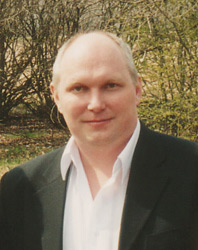Reactionless Propulsion and Active Force

Abstract
The goal of the report is to demonstrate some technological ways to create active force that don't require reactive mass flow. The active force is applied to many aerodynamics systems and it is result of the pressure difference from the medium to the system. By analogy, the space-time itself can be considered as some medium of interaction.

Introduction

There are known mechanical systems of gyroscope precession, gyroscopes of alternating radius and gyroscopes of nonlinear vibrations. For other systems the velocity of rotation of the mass is changing for different parts of the trajectory that produce some inner gradient of pressure in the system from the rotor to the stator. Some mechanical systems use special vortex.

In some electromechanical systems electrically charged rotor is used to create propulsion force.

There are known electromagnetic systems to use Lorenz force, magneto-hydro-dynamical effect, Hall current. Some antigravitation effects are investigated for so called Hooper bi-directional coils and in the flat current systems (Mobius loop). Quite old idea is to use diamagnetic vapor that is accelerating in the permanent magnet field.

High electric potential systems are known by Tesla and T.T.Brown research work. The Biffeld-Brown effect describes the active force that is created in any flat capacitor to the positive plate side for voltage above 50 kV. Dr. Brown did not explanation of the effect to find the reason of the force so we have chance to suppose that this force is result of the asymmetry for orbital motion of the electrons in the dielectric atoms in strength of the electric field between plates. The asymmetry lead to centrifugal force gradient and non-zero linear component of this force.

There are some research program on superconductivity – gravitation connection.

Special case is thermo-gravitation propulsion technologies. Fig.1.1 show plan for experiment that was described by Alexander Shegolev, Russia as weight changes in heated metal ball if in the top of the ball the cone part was removed to create non-zero total heat flow of this mass.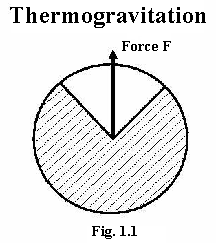Let's find the reasons for this active (reactionless) force.

It is known that interaction between atom or molecule and space-time demonstrated as chaotic
oscillations or movements those are considered as heat processes in matter. In material objects of
special shape (like the ball above) the vector sum of such sort oscillations is not equal to zero so the object get some impulse in the direction that is defined by its shape. The nature of this impulse is the same like the nature of chaotical impulses those are received by any atoms from the space-time (from the ether).

Fig.1.2. show plan for another thermogravitation closed system design: the reactive flow is
transformed by some converter. One of many versions for it is the cooling of the flow by means of
special vortex process. Like for Fig.1.1  the active force is result of non-zero vector sum for heat processes of each particle of the matter.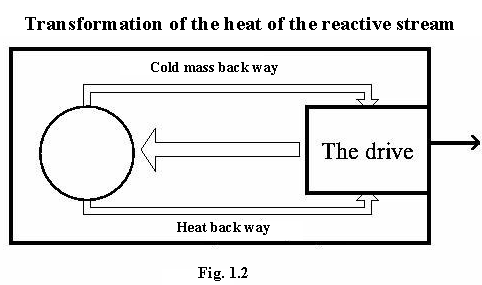To continue we have to make some supposition about the nature of the heat energy. According to Pavel D. Ouspensky (Russian philosopher) and Nikolay A. Kozyrev (Russian astrophysics) there is energy exchange between particle of matter and so called "flow of time" for any really existing material particle. In other words, it is the physical mechanism of existence of the mass-matter in the time flow. By P.D.Ouspensky the heat chaotical oscillations are the result of this energy exchange. By N.A.Kozyrev, the stars have not any inner source of energy but they are working like some kind of machine that transform the energy of so called "flow of time" into the energy of heat radiation (electromagnetic waves). So, we can make the conclusion: The existence of any material system is defined by its energy exchange with so called "flow of time" of certain velocity that is rate of the process.

Modern physics of vacuum consider the notion about so called "virtual particles". Old idea for the ether particles also can explain chaotical impulse interaction between real particle of matter and vacuum (ether). The target now can be formulated by next way: active drive should be designed if the conditions for directed impulse energy exchange between real and virtual particles are created. Such sort interaction takes place anytime and always but total vector sum is zero in strength of spontaneous nature of the process.
We have chance to order this process as directed heat flow in the mass of the drive, or to influence onto vacuum by means of force field (electrical, for example) to order the processes of creation and annihilation of the virtual particles. Such sort influence is the reason of electrical interactions. Fig.2.1 show the repulsion and Fig.2.2. show the attraction modes for two electrically charged bodies.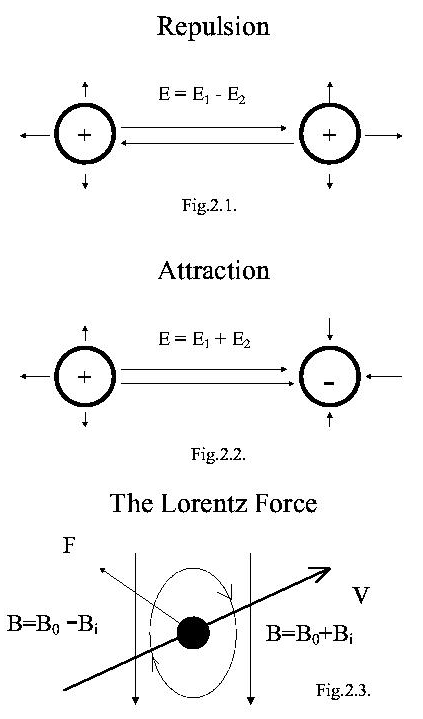The first case, total sum intensity between bodies is less than intensity of each body. The second case, the intensity between bodies is more than intensity of electrical field of each body. In fact, each electrically charged body is surrounded by joint summary electrical field that is not symmetrical and some gradient of electrical intensity is the reason for force. It is important to understand that there is direct interaction between body and surrounding space (ether) and force is result of the gradient of the pressure like for aerodynamics. There is no needs in hypothesis about so called "long distance interaction" since all force interactions (electrical included) are simple mechanical effects in the ether.

Lorenz force also can be considered as result of some gradient in the ether, Fig.2.3. since the pressure of the ether depend of the relative velocity (vector sum or difference) . The effect seems as the aerodynamics but for the ether case.

Since the "intensity of the field" itself is the gradient of the 'potential" ( E = - grad phi ) so the gradient of the "intensity of the field" (grad E) is quality new notion that lead to new physical effect, i.e. to the active non compensated force.

Assuming the common nature of the considered examples we have to conclude: the base for
electrical and magnetic interactions is the same like for energy exchange of heat processes. The
"field" means some area of space where the processes of interaction between the ether and matter are not chaotical and spontaneous but they are ordered in some degree. More order means more value of the force. The field influence to processes of impulse interaction between material particle and ether (virtual) particle to make this interaction directed in some certain direction. By this way we are coming to the mechanics on the ether level as the base for electricity and gravitation that is electrogravitational unification.

Let's consider some design, where the properties of the matter are used to create the gradient described above. It is necessary note that there is no difference in principle between particle of matter and space area near of the particle that was mentioned by Faraday. So, when we are working to make some special structure of the matter, we are working with space structure in the volume of this material object.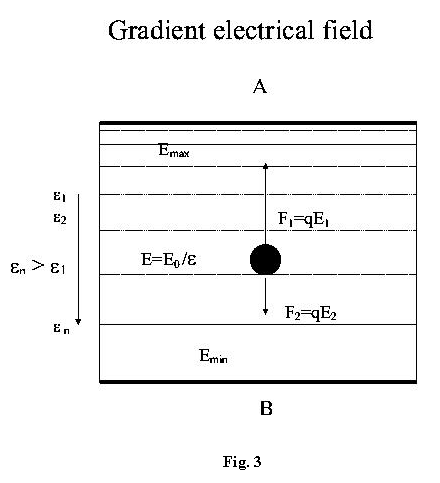The dielectric, Fig.3. is placed between plates is non-linear since there is gradient of permittivity along the electrical intensity lines. Normally, the polarization of particles of the dielectric is symmetrical in positive and negative plate side. But in this case, the asymmetry of properties of the dielectric produces the asymmetry of polarization and there is some non-compensated force in such sort capacitor. T.T.Brown patented something like this in USA patent 3187206 of May 9, 1959. Simplest case is two-layers dielectric, Fig.4.1. where the force is created on the border between two different dielectrics. This force is known from 1927 and it is applied for industry as Johnsen-Raabek effect.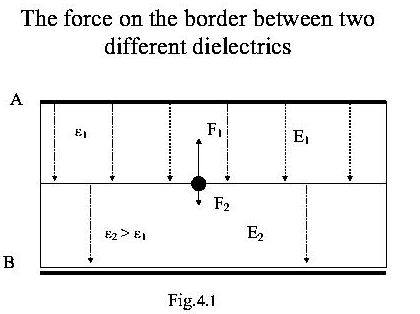Let's consider some experiment, Fig.4.2. The base of the design is dielectric of permittivity 6 and second layer is dielectric of permittivity 1. The square of the plate is 100 square cm. The high potential direct current source is self-made design. The potential difference is about 10 kV. The calculated active force should be about 0.001 N or 150 mg that was detected by means of rotation torsion effect.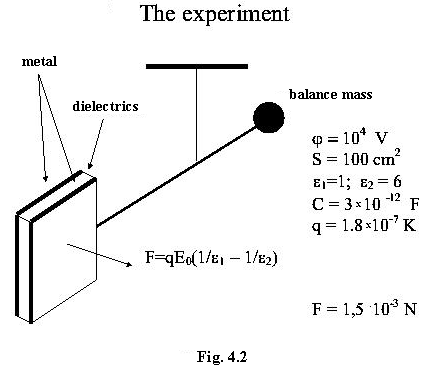Fig.5 is approximated calculation for commercial project. The surface is taken as 100 square meters. The capacity of sphere for 1 cm radius is 1 pF, the calculated capacity should be about 1 micro Farad. The special ceramics can be used that allow increase the permittivity from 1 up to 80. The potential of 100 kV is quite real value. So, for this potential and the surface we'll get the electrical charge about 8 Coulomb. The difference of forces those are acting on the particles of this gradient dielectric is about 80 ton.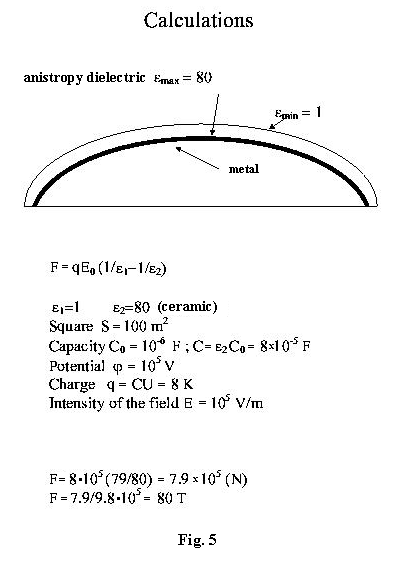There is square function for force from the potential, so it is more useful to increase the potential for the same surface than to increase the surface.

In the conclusion let me say about necessity for true law of impulse conservation. The name of the report "reactionless propulsion" noted the absence of ordinary reactive flow of fuel mass. In the systems are considered above there is the reaction since the velocity of system is changing and the compensation effect here is the change of the impulse of the "virtual particles" or "particles of the ether". So, the heat energy exchange balance should be changed and the ether becomes colder. We can detect it since the time rate should be changed for the mass of so called "working body" of such sort active drive.

Alexander V. Frolov
June 20, 1998. St.-Petersburg

The paper was presented as the report on International Scientific Congress "Space, Time and Gravitation", St.-Petersburg, 1998.

At first time short version of the paper was published in ELECTRIC SPACECRAFT,  Leicester, North Carolina 28748 USA, Issue 27,  1997 p.30-31.